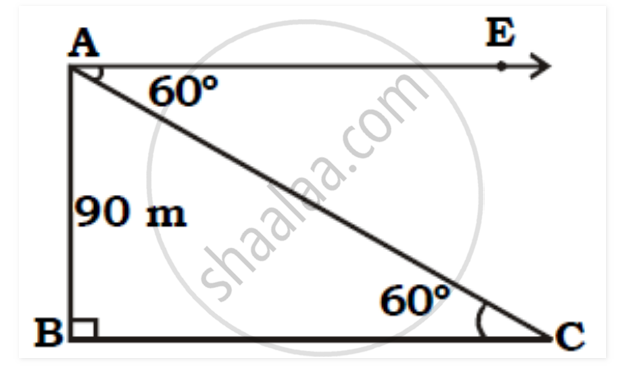# From the Top of a Lighthouse, an Observer Looks at a Ship and Finds the Angle of Depression to Be 60° . If the Heiht of the Lighthouse is 90 Meters, Then Find How Far is that Ship from the Lighthouse? - Geometry

Sum

From the top of a lighthouse, an observer looks at a ship and finds the angle of depression to be 60° . If the height of the lighthouse is 90 meters, then find how far is that ship from the lighthouse? (√3 = 1.73)

#### SolutionAs shown in the figure, assume AB as the lighthouse and let A be the position of the observer and C be the position of the ship. Let the distance from the ship to the lighthouse be x.

Let AB be the height of the lighthouse,

∴ AB = 90 metres [Given]

The point 'C' be the position of the ship,

∴ ∠ ACB = 600

tan 600 = Opposite side of 600 / Adjacent side of 600

∴ tan 600 = AB / BC

∴ √3 = 90/BC

∴ BC = 90/√ 3

∴ BC = (90/√3)× √3/√3

∴ BC =  30√3

∴ BC = 30× 1.73

∴ BC = 51.9 m.

∴ The ship is 51.9 m away from the lighthouse.

Concept: Heights and Distances
Is there an error in this question or solution?